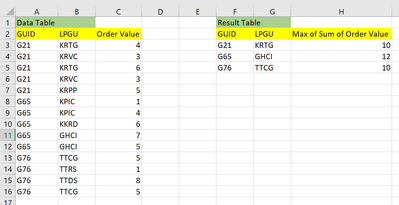cancel
Showing results for
Did you mean:Anonymous
Not applicable

## Filtering Table ValuesHello All, @parry2k @smpa01 @AlexisOlson

I wish to create a Result Table from the Data Table as seen in the attached screenshot. The Result Table must be such that it will show only those values in the three columns which are corresponding to the Maximum of Sum of Order Value.

It will be helpful if we can achieve this functionality.

Thanks

1 ACCEPTED SOLUTIONSuper User

@Anonymous  this will be table expression

``````Table 2 =
var _grp = GROUPBY('Table','Table'[GUID],'Table'[LPGU],"subTotal by GUIDLPGU",SUMX(CURRENTGROUP(),'Table'[Order Value]))
--var _filt = TOPN(1, FILTER(_grp,var _guid = [GUID] return [GUID]=_guid),[x],DESC)
var _rank = SUMMARIZE(FILTER(ADDCOLUMNS(_grp, "rank", RANKX(FILTER(_grp,[GUID]=EARLIER([GUID])),[subTotal by GUIDLPGU],,DESC)),[rank]=1),[GUID],[LPGU],[subTotal by GUIDLPGU])
return _rank``````

@AlexisOlson  in a table expression is it possible to ask TOPN to return TOPN(1) based on a partiton like in RANKX

``RANKX(FILTER(_grp,[GUID]=EARLIER([GUID])``

I tried but failed.

Proud to be a Super User!

New Animated Dashboard: Sales Calendar

12 REPLIES 12Super User

Assuming you have a measure for the sum of Order Value

``SumOrderValue = SUM ( Table1[Order Value] )``

then we can write a measure for the top LPGU order fairly neatly:

``````Top LPGU Order =
VAR TopLPGU =
MAXX ( TOPN ( 1, ALLSELECTED ( Table1[LPGU] ), [SumOrderValue] ), Table1[LPGU] )
RETURN
CALCULATE ( [SumOrderValue], KEEPFILTERS ( Table1[LPGU] = TopLPGU ) )``````

Edit: You can easily make this into a calculated table as follows:

``SUMMARIZECOLUMNS ( Table1[GUID], Table1[LPGU], "Order Value", [Top LPGU Order] )``Super User

@Anonymous  you can write a measure like this

``````_Measure =
CALCULATE (
CALCULATE (
SUM ( 'Table'[Order Value] ),
ALLEXCEPT ( 'Table', 'Table'[GUID], 'Table'[LPGU] )
),
KEEPFILTERS (
TOPN (
1,
FILTER ( ALLSELECTED ( 'Table' ), 'Table'[GUID] = MAX ( 'Table'[GUID] ) ),
CALCULATE (
SUM ( 'Table'[Order Value] ),
ALLEXCEPT ( 'Table', 'Table'[GUID], 'Table'[LPGU] )
), DESC
)
)
)``````Proud to be a Super User!

New Animated Dashboard: Sales CalendarAnonymous
Not applicable

@smpa01 This looks good. Can we implement this as Calculated Table with all 3 columns?Super User

@Anonymous  this will be table expression

``````Table 2 =
var _grp = GROUPBY('Table','Table'[GUID],'Table'[LPGU],"subTotal by GUIDLPGU",SUMX(CURRENTGROUP(),'Table'[Order Value]))
--var _filt = TOPN(1, FILTER(_grp,var _guid = [GUID] return [GUID]=_guid),[x],DESC)
var _rank = SUMMARIZE(FILTER(ADDCOLUMNS(_grp, "rank", RANKX(FILTER(_grp,[GUID]=EARLIER([GUID])),[subTotal by GUIDLPGU],,DESC)),[rank]=1),[GUID],[LPGU],[subTotal by GUIDLPGU])
return _rank``````

@AlexisOlson  in a table expression is it possible to ask TOPN to return TOPN(1) based on a partiton like in RANKX

``RANKX(FILTER(_grp,[GUID]=EARLIER([GUID])``

I tried but failed.

Proud to be a Super User!

New Animated Dashboard: Sales CalendarSuper User

I'm not quite sure what you mean but you can simplify your _rank variable to be:

``````FILTER (
_grp,
RANKX (
FILTER ( _grp, [GUID] = EARLIER ( Table1[GUID] ) ),
[subTotal by GUIDLPGU]
) = 1
)``````

or like this

``````TOPN (
1,
_grp,
RANKX (
FILTER ( _grp, [GUID] = EARLIER ( Table1[GUID] ) ),
[subTotal by GUIDLPGU]
), ASC
)``````Super User

@AlexisOlson Thanks.  I was not asking you about RANKX. I was wondering if it is possible to pass on a partitoned table in TOPN like I did in RANKX?

Can I pass on a similar table like following in TOPN, so that TOPN (1) gives me thre result for that partiton?

``````RANKX (
FILTER ( _grp, [GUID] = EARLIER ( Table1[GUID] ) )``````

Proud to be a Super User!

New Animated Dashboard: Sales CalendarSuper User

Sorry, I'm still not sure I understand what you mean by "pass a partitioned table".

If you try to write this

``TOPN ( 1, FILTER ( _grp, [GUID] = EARLIER ( Table1[GUID] ) ), [...] )``

it just doesn't make any sense because there's no earlier row context to refer to.Super User

@Anonymous , Based on what I got, a measure to be used with GUID, LPGU in visual

sumx(summarize(Table, Table[GUID], Table[LPGU], "_sum", sum(Table[Order Value] ) ), [_sum])Anonymous
Not applicable

Seems that doesn't work as it still fetches multiple values of LPGU, instead it must fetch single values of GUID, LPGU corresponding to Max Sum of Order Value as shown in the Result Table. Also I need to create Result Table as Data Table(not visual table).Super User

@Anonymous , Try like

sumx(summarize(Table, Table[GUID], "_sum", sum(Table[Order Value] ) ), [_sum])

If this does not help
Can you share sample data and sample output in table format? Or a sample pbix after removing sensitive data.Anonymous
Not applicable

@amitchandak This too doesn't work and I still can see multiple values of LPGU, GUID and Order Value.

It needs to go like Result Table Must show filtered GUID, LPGU and Maximum of Sum of Order Value and that Sum of Order Value must be done by LPGU.

Unfortunately, I can't present the sample data. You Can take data from the screenshot that I attached. Also keeping the data table here.

 GUID LPGU Order Value G21 KRTG 4 G21 KRVC 3 G21 KRTG 6 G21 KRVC 3 G21 KRPP 5 G65 KPIC 1 G65 KPIC 4 G65 KKRD 6 G65 GHCI 7 G65 GHCI 5 G76 TTCG 5 G76 TTRS 1 G76 TTDS 8 G76 TTCG 5Anonymous
Not applicable

@amitchandak I'll check now and get back soon. Thanks